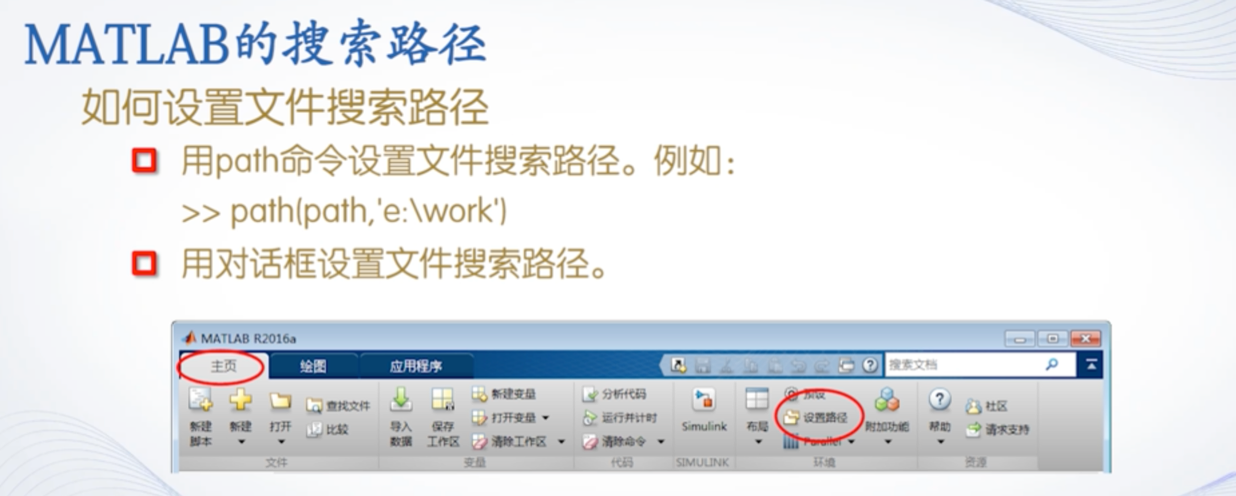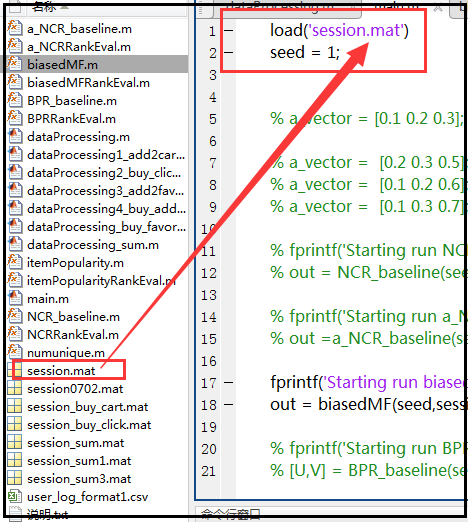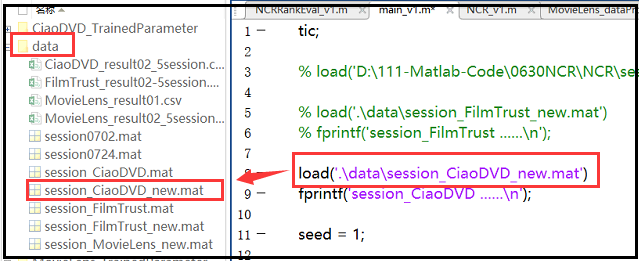• 使用通配符在 MATLAB 搜索路径中搜索 WHICHX 文件 命令窗口中的输出是链接的，因此可以直接在编辑器或 GUIDE 中启动 M-Files，打开 MDL-、FIG-files 和其他类型。 请看截图。 例子： --- WHICHX *.m 列出了 matlab ...
•展开全文• which函数可以给出指定文件的路径 ...（1）当要查找的文件路径中，三层及以上的母文件夹名字中有大写字母时，它的输出路径中会将大写字母变成小写字母，比如用which查找ccc的路径，它会输出：E:\ toolbox\ matlab...
which函数可以给出指定文件的路径
用法： which（‘aaa’）（查找文件名为aaa的文件的路径） 比如输出路径为：E:\ toolbox\ matlab\ myDIYfile\ aaa.m
需要注意： （1）当要查找的文件路径中，三层及以上的母文件夹名字中有大写字母时，它的输出路径中会将大写字母变成小写字母，比如用which查找ccc的路径，它会输出：E:\ toolbox\ matlab\ mydiyfile\ a\ b\ ccc.m其中文件夹myDIYfile已经变成mydiyfile。 （2）在matlab中，并不区分路径中的大小写字母，比如 输入命令 cd E:\ toolbox\ matlab\ mydiyfile\ a\ b 和命令cd E:\ toolbox\ matlab\ myDIYfile\ a\ b都会进入到b这个文件夹中。 （3）但是，当将两个路径作为字符串进行对比时，比如 strcmp (which(‘ccc’)，‘E:\ toolbox\ matlab\ myDIYfile\ a\ b\ ccc.m’)，则结果为false，因为which(‘ccc’)会将大写DIY变成diy，那么用字符串来对比路径就不明智了。
附加： 如果想得到aaa.m文件所在的文件夹路径可以用fileparts函数： dir1=which aaa; [dir2, ~]= fileparts(dir1); %dir1为文件aaa的路径 E:\ toolbox\ matlab\ myDIYfile\ aaa.m %dir2为aaa所在文件夹的路径 E:\ toolbox\ matlab\ myDIYfile
展开全文• fileFolder=fullfile(matlabroot,'mathclass','raccoon');%遍历文件夹下所有符合条件的文件 dirOutput=dir(fullfile(fileFolder,'ra*.jpg'));%提取路径 fileNames={dirOutput.name}';%获得符合条件文件名 Mat
1、带后缀
fileFolder = fullfile(matlabroot,'mathclass','raccoon');    % 遍历文件夹下所有符合条件的文件
dirOutput = dir(fullfile(fileFolder,'ra*.jpg'));   % 提取路径

fileNames = {dirOutput.name}';   % 获得符合条件文件名
Matlab dir命令

Matlab使用dir函数获得指定文件夹下的所有子文件夹和文件,并存放在在一种为文件结构体数组中.

dir函数可以有调用方式为：
* dir('.')列出当前目录下所有子文件夹和文件
* dir('G:\Matlab')列出指定目录下所有子文件夹和文件
* dir('*.m')列出当前目录下符合正则表达式的文件夹和文件

2、不带后缀

global pathname  imname;

%fileneme 指的就是带文件后缀的文件名，如‘050.bmp’

%pathname指的是文件路径名

[filename, pathname] = uigetfile('*.bmp', 'Input filename');

%寻找后缀名前面的标志‘.’

i = find('.'==filename);

%去除文件后缀，提取单纯的文件名

imname = filename(1: i-1);

fid1 = fopen(strcat(pathname, imname, '.bmp'),'r');

%判断文件是否已经被打开

if(fid1 == -1)
msgbox('Input File or Path is not correct', 'Warning', 'warn');
return;
end
I2 = I1(:,:,1)/3 + I1(:,:,2)/3 + I1(:,:,3)/3;
I = imshow(I2);

参考：

http://www.cnblogs.com/cherler/p/3727713.html
http://blog.csdn.net/shenziheng1/article/details/50933435


展开全文2、文件在下一级路径下： 使用  load (' .\下一级路径的文件名\***.mat ');3、文件在上一级路径下： 使用  load (' ..\***.mat ');

转载于:https://www.cnblogs.com/shenxiaolin/p/9381878.html
展开全文• Matlab下有时命令出错，源于Command窗口的路径不正确。快捷键的执行会受此影响。 转载于:https://www.cnblogs.com/zdwt/p/9744101.html
• 首先在m脚本文件canshuo.m中输入节点个数和路径权重 在命令窗口中输入canshu 用s=12,e=10的格式输入要求的起止点，再输入main即可得到两点之间的路径和长度。
• 最近在做一个变化检测的实验，老师给我了一个代码，里面的许多读取文件都是采用的绝对路径，类似如下： 改来改去的很麻烦，所以查找了一些关于matlab读取文件的命令，想用... 这个命令会将文件路径自动切割为四个部...
• ## MATLAB路径设置

千次阅读 2018-06-04 15:17:48
Matlab的搜索路径MATLAB搜索路径的添加使用图形用户界面添加搜索路径使用命令添加搜索路径添加绝对路径添加相对路径MATLAB搜索路径的移除移除单个路径移除多个路径还有一种方法Matlab的搜索路径搜索路径（search ...
• '‘当前工作目录’'也称为当前目录或当前文件夹，指的是matlab用来查找、打开、保存文件的位置，下图所示的就是当前工作目录。 1.查看当前工作目录： >> pwd %(print current working directory)显示当前...
• MATLAB App Designer编译打包exe后存在找不到文件路径或调用文件出错的问题，可以使用pwd命令app
• ## matlab处理中文路径

千次阅读 2018-02-23 10:50:30
• matlab一开始打开的文件路径可能不是自己要的，可以通过方式进行修改： 方法一：通过命令行 在matlab里面通过以下命令进行修改： userpath('想要更换成的路径') savepath 这样就修改完成了，可以通过matlabpath...
• 这时，matlab文件目录和文件的操作有着重要的作用。 一、 matlab路径的操作 1、 filesep 用于返回当前平台的目录分隔符，Windows是反斜杠(\)，Linux是斜杠(/)。 2、 fullfile 用于将若干字符串连接成函数
• 参考： ...我们知道，在matlab里添加路径和删除路径可以直接使用【设置路径】这一个选项添加路径和删除路径。但是这样操作的缺点就是，1、对路径的影响是永久性的；2、无法...
• 命令窗操作1.命令窗的显示2.数据显示格式3.命令行的标点符号4.命令窗常用控制命令5.指令行编辑二.当前文件夹和路径设置1.当前文件夹及其使用2.搜索路径路径设置三.工作内存区和历史命令窗1.工作内存区、交互绘图2....
• 1.MATLAB系统由五大部分构成： MATLAB开发环境、 MATLAB数学函数库、 MATLAB语言、 MATLAB图形处理系统 MATLAB应用程序接口（API）。 2.MATLAB系统提供了两个重要部件：Simulink和Toolboxes。 3.换行时，只要在...
• matlab无法识别当前路径下的文件 现象：m文件中应用的某个函数给出警告：xxx是无效变量或函数 解决方案： 有可能当前路径下确实没有警告中所说的文件，需要包含相应文件matlab路径搜索规则：若子文件夹下含有...
• 我们到过那里...您知道您在某个地方写了一些代码可以帮助您完成任务，但是您无法... （i）在MATLAB编辑器中打开m文件，然后（ii）将“当前文件夹”更改为匹配的m文件的目录。 在代码搜索的帮助文本中描述了其他功能。
• 通常m文件的默认搜索路径是在work文件夹下，如果想自己设置一个文件夹用来存储m文件，会提示不在路径。可以直接点提示窗口的添加到路径。 设置方法： 主页-环境-预设路径，将你的路径添加进去 ...
• 这个工具是为通过 MATLAB 命令提示符简单、快速和肮脏的 git 使用而设计的。 除此之外没有任何承诺。 如果您需要更多功能，请查看此链接： ...
• function,创建函数文件： function [ VecFolders ] = FindFolders( InputDir,IsReturnNameOnly ) %查找当前路径下所有文件夹 %查找当前路径下所有文件夹 %InputDir: 输入路径 %IsReturnNameOnly：是否只返回文件夹...
• matlab第一次运行m文件就遇到了这样的问题。
• 默认情况下，Matlab软件一打开就...需要注意的是：若两种都修改，必须路径一致，否则matlab无法识别具体路径，会继续打开默认路径 右键点击matlab软件图标，在点击属性，在【起始位置】框里输入你所需要的路径即...
• MATLAB® 搜索路径文件系统中所有文件夹的子集。MATLAB 使用搜索路径来高效地定位用于 MathWorks® 产品的文件。搜索路径上的文件夹顺序十分重要。当在搜索路径上的多个文件夹中出现同名文件时，MATLAB 将使用...classpath
• 它打算在MATLAB命令窗口中使用。 安装： 从 MATLAB Central File Exchange 下载完整的 zip 文件，在您的 MATLAB 路径上的任意位置解压并输入 jgit。 这将下载最新版本的 org.eclipse.jgit 并在 MATLAB 首选项目录...
• 单击matlab主界面菜单工具栏中的“设置路径”按钮，打开“设置路径”对话框，左侧的几个按钮用来添加目录到搜索路径，还可以从当前的搜索路径中移除选择的目录，右侧的列表框列出了已经被matlab添加到搜索路径的目录...
• Matlab:为什么文件明明在搜索路径中，Matlab却说找不到呢？ 你应该是把文件放在matlabroot/toolbox路径下了吧，如果是这样，这个问题嘛，就是由于Matlab为了提高启动和运行速度所采取的一个优化措施有关。Matlab把......

# matlab命令文件路径matlab 订阅